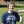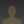0%
0%

# FPGA Frankenstein as CW Transmitter

Radio telegraph(cw) transmitter based on the Frankenstein FPGA evaluation board

Similar projects worth following
537 views
Using the FPGA I did last time, I will make a radio transmitter.

To make a transmitter is very simple!

If we take a certain frequency generator and connect it to the antenna, we get a simple transmitter.

(We are not going to consider the problems now: load matching, the wave resistance of the communication line, the resonant frequency of the antenna.)

After that, if we have a receiver on that frequency and modulation, we will be able to receive the signal. At short distances, the receiver can receive clock generators even from a conventional Board with a microcontroller.

Let's use this hack and create a simple Telegraph transmitter!

PS. Number of micro-cells occupied by the project — 30 out of 64.

PPS. You can help improve the quality of the English text in this project. Please write message to me.

### epm7064_cw_tx.rar

Altera Quartus Project

RAR Archive - 10.32 kB - 11/21/2018 at 14:46

• 1 × FPGA boaed based on Altera EPM7064 For generate radio signal
• 1 × RTL SDR R820T2 Receiver For receive in CW mode
• 1 × The cut of the wire as an antenna

• 1
Telegraph. Theory.

If you just turn on the transmitter (generator) at a certain frequency, it will not transmit any useful information. To transmit information, the radio signal must to be modulated. There are many different kinds of modulation.

In HAM-radio practice, the simplest mode of communication is Telegraph (CW). Telegraph is a unique type of communication. Telegraph can be considered the first digital form of communication. However, a trained person is able to decode this digital signal by ear. Now to decode the Telegraph signal can be used decoder software. But the human ear is still considered to be the most accurate instrument for receiving a Telegraph signal. And also the telegraph is one of the most "long-range" types of communication. Due to the transmission at one frequency and low transmission speed, all signal energy is concentrated on one frequency, it has a positive effect on the transmission distance. Narrowband filters can be used for reception. A Telegraph signal consists of dots and dashes. Each character in the Telegraph alphabet is a combination of dots and dashes. The most commonly used letters consist of fewer elements.

Dots and dashes are the facts of turning on the transmitter. In the intervals between the dots and the dash, the transmitter turns off, the signal is not transmitted to the air. There are rules by which the dash must have a duration equal to three dots. The space between dots or dashes in one character must be equal to the duration of one dot. The space between the letters in one word must be three dots. The space between words must be at least seven dots.

If we connect a short-length wire (as an antenna) to the FPGA pin and send a clock signal to this pin, we will be able to receive this signal as an unmodulated carrier frequency. Turning on or off the signal to the antenna, we will modulate the carrier. And forming the correct time intervals for dots and dashes, we will form a Telegraph radio signal.

• 2
Telegraph signal generation

To form time intervals in fractions of a second, we need to divide the clock signal. In my case, the frequency of the clock generator is equal to 25175000 Hz. I decided to take a 23 bit binary counter. The frequency obtained as a result will be equal to:

```the counter is 2^22 = 4194304 (steps)
clock frequency 25175000 Hz
result frequency 25175000 / 4194304 = 6 Hz```

The resulting frequency of 6 Hz is sufficient to form a single dot interval. Now let's try to form something very simple. For example, SOS signal: three dots, three dashes and three dots. Draw a time diagram:

From the diagram we can see that we need 30 steps. 30(dec) = 11110(bin). That's 5 bit's. That is, we add another 5 bits to the 22 bits of the counter. And we get 27 bits.

Now the logic is very simple (because complex logic we can not put in such a small FPGA): when the value of the counter is equal to 0, 2, 4, 8, 9, 10... (and forth on diagram) we are set 1, otherwise we set 0. This signal (CW) can already be output from the FPGA and controlled, for example, by flashing the led. And to modulate the radio signal, we will output the clock signal to the output only if the value of the CW signal is equal 1.

```module epm7064_test(clk, out_lf, out_rf);
input wire clk; // clock input
output wire out_lf; // low frequency cw output
output wire out_rf; // modulated radio frequency cw output

reg [27:0] cnt; initial cnt <= 28'd0;

always @(posedge clk) cnt <= cnt + 1'b1;

// 5 higth bits of the counter for generate symbols
wire [4:0] hi_bits = cnt[26:26-4];

wire cw = (hi_bits == 5'd0) ||
(hi_bits == 5'd2) ||
(hi_bits == 5'd4) ||
(hi_bits == 5'd8) ||
(hi_bits == 5'd9) ||
(hi_bits == 5'd10) ||
(hi_bits == 5'd12) ||
(hi_bits == 5'd13) ||
(hi_bits == 5'd14) ||
(hi_bits == 5'd16) ||
(hi_bits == 5'd17) ||
(hi_bits == 5'd18) ||
(hi_bits == 5'd22) ||
(hi_bits == 5'd24) ||
(hi_bits == 5'd26);

assign out_lf = cw;
assign out_rf = cw & clk;

endmodule
```

That's all, it's time to turn on the receiver!

• 3

What is the receiver suitable for the reception of the Telegraph? To receive a Telegraph, you need a single-band receiver. When I chose the frequency of the transmitter, I cheated a bit. I have a USB SDR receiver, the lower limit of reception is 24 MHz, so the frequency of the generator I chose taking into account that the signal can be taken to this receiver. This receiver can be bought on ebay/aliexpress is very cheap. Search for words R820T2. As a program for receiving I am using the SDRSharp.

Share

## Similar Projects

Project Owner ContributorPhil Handley

Project Owner Contributor

### FPGA + 3 R + 1 C = MW and SW SDR ReceiverAlberto Garlassi

# Does this project spark your interest?

Become a member to follow this project and never miss any updates